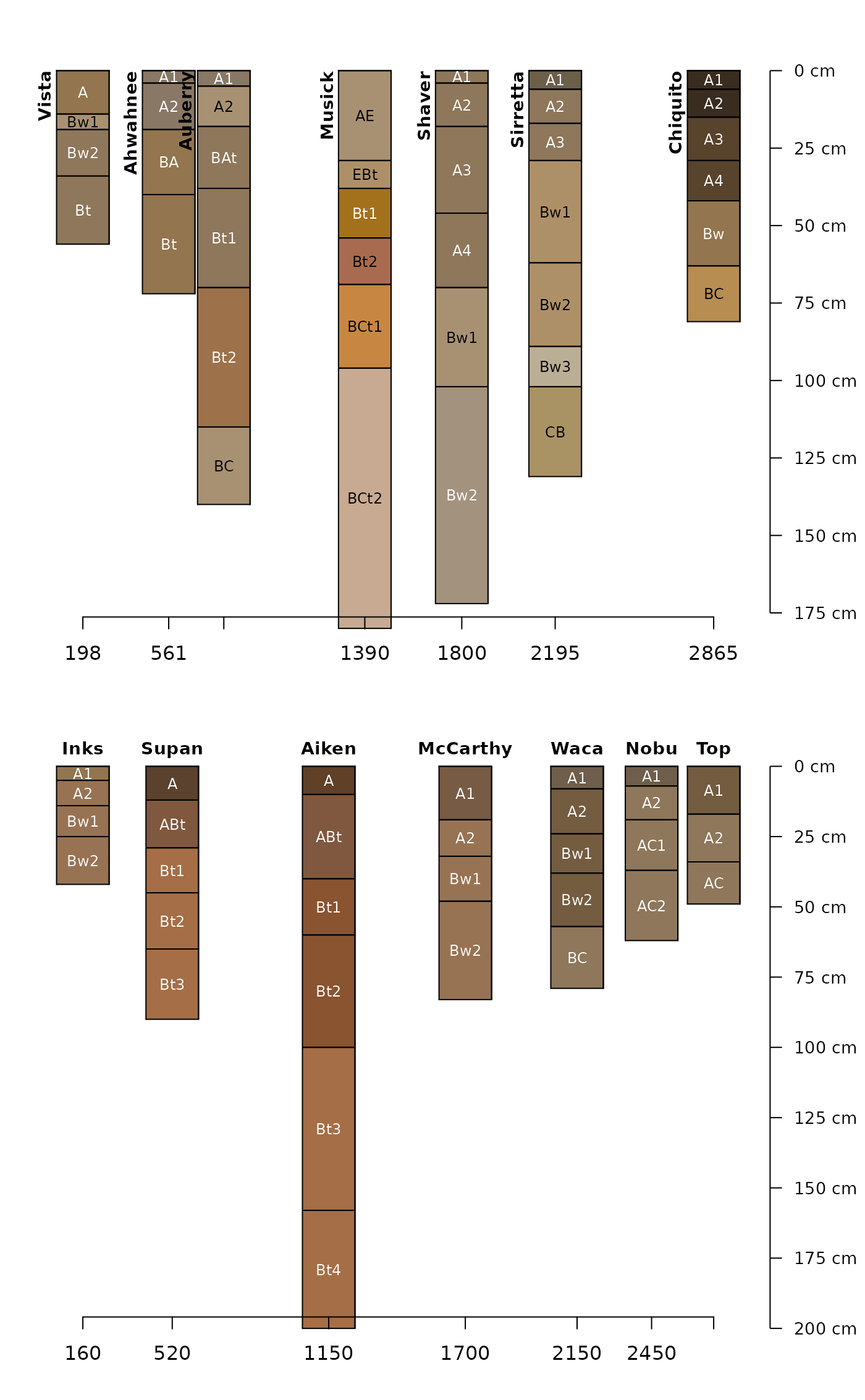This function is used to support relative positioning of soil profiles by plotSPC, based on transect or gradient values typically associated with a site level attribute (e.g. elevation). Gradient values specified in x are translated to the range used by plotSPC (usually 1, length(SPC)) specified in x.min and x.max.

alignTransect(x, x.min, x.max, fix = TRUE, ...)

## Arguments

x

numeric vector, describing values along a transect: distance, elevation, climatic variables, etc.. Typically sourced from the site level attributes of a SoilProfileCollection object. Order is not important.

x.min

numeric, lower boundary to relative position scale

x.max

numeric, upper boundary to relative position scale

fix

logical, attempt fixing overlapping positions with fixOverlap

...

additional arguments to fixOverlap

## Value

list containing:

• grad: values of x in ascending order

• order: ordering vector of x

• relative.pos: elements of x translated to the new relative scale defined by x.min and x.max

## Details

See the Pair-Wise Distances by Generalized Horizon Labels tutorial for additional examples.

## Examples


data("sierraTransect")

# split transects
g <- subset(sierraTransect, transect == 'Granite')
a <- subset(sierraTransect, transect == 'Andesite')

g.p <- alignTransect(gelev, x.min = 1, x.max = length(g), fix = FALSE) a.p <- alignTransect(aelev, x.min = 1, x.max = length(a), fix = FALSE)

op <- par(mar=c(2,0,0,2), mfrow=c(2,1))

plotSPC(g, width=0.25, name.style='center-center',
cex.names=0.75,
relative.pos = g.p$relative.pos, plot.order = g.p$order)

axis(1, at = g.p$relative.pos, labels = g.p$grad, line = -1.5)

plotSPC(a, width=0.25, name.style='center-center',
cex.names=0.75,
relative.pos = a.p$relative.pos, plot.order = a.p$order)

axis(1, at = a.p$relative.pos, labels = a.p$grad, line = -1.5)par(op)